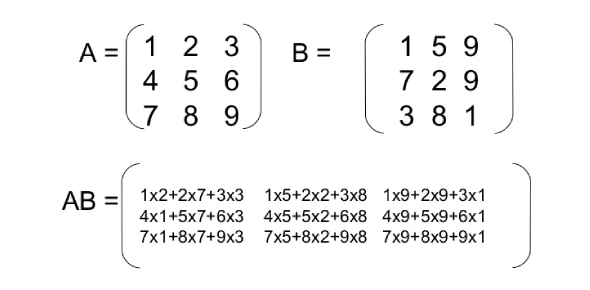Multiplication Ultimate Math Quiz: Test!

25 Questions | Total Attempts: 163SettingsMultiplication is one of the four elementary math operations of arithmetic. It is in the same grouping as division, subtraction, and addition. Children learn early in school how to multiply numbers and solve equations. The result of a multiplication is called products. If you want to know more about multiplication, then this is the quiz for you.

• 1.
10x2=
• 2.
10x3=
• 3.
10x5=
• 4.
10x0=
• 5.
0x10=
• 6.
10x1=
• 7.
1x10=
• 8.
2x10=
• 9.
3x10=
• 10.
10x4=
• 11.
4x10=
• 12.
5x10=
• 13.
10x6=
• 14.
6x10=
• 15.
10x7=
• 16.
7x10=
• 17.
10x8=
• 18.
8x10=
• 19.
10x9=
• 20.
9x10=
• 21.
10x10=
• 22.
10x11=
• 23.
11x10=
• 24.
10x12=
• 25.
12x10=
Related TopicsBack to top